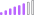cancel
Showing results for
Did you mean:

Fabric is Generally Available. Browse Fabric Presentations. Work towards your Fabric certification with the Cloud Skills Challenge.Post Patron

## Check if client id exists in current year and doesn't exist in previous year

Hi,

I have a requirement to find if the client exists in current year but doesn't exist in previous year.

How do I achieve this?

1 ACCEPTED SOLUTIONCommunity Support

Hi, @bml123

I simply simulated some data that would solve your problem in 2 ways.20 and 30 are client ids that exist in this year and did not exist in the previous year.

Method 1:

Measure:

``````Measure =
Var N1=CALCULATE(COUNT('Table'[client id]),
FILTER(ALL('Table'),[client id]=SELECTEDVALUE('Table'[client id])&&YEAR([Date])=YEAR(TODAY())))
Var N2=CALCULATE(COUNT('Table'[client id]),
FILTER(ALL('Table'),[client id]=SELECTEDVALUE('Table'[client id])&&YEAR([Date])=YEAR(TODAY())-1))
return
IF(N1>0&&N2=BLANK(),1,0)``````Method 2:

Table:

``````Table 2 =
Var table1=Filter(SUMMARIZE('Table','Table'[client id],'Table'[Date]),YEAR([Date])=YEAR(Today()))
Var table2=Filter(SUMMARIZE('Table','Table'[client id],'Table'[Date]),YEAR([Date])=YEAR(Today())-1)
return
Except(SUMMARIZE(table1,'Table'[client id]),SUMMARIZE(table2,'Table'[client id]))``````Is there a way to output the results you expect?

Best Regards,

Community Support Team _Charlotte

If this post helps, then please consider Accept it as the solution to help the other members find it more quickly.

3 REPLIES 3Community Support

Hi, @bml123

I simply simulated some data that would solve your problem in 2 ways.20 and 30 are client ids that exist in this year and did not exist in the previous year.

Method 1:

Measure:

``````Measure =
Var N1=CALCULATE(COUNT('Table'[client id]),
FILTER(ALL('Table'),[client id]=SELECTEDVALUE('Table'[client id])&&YEAR([Date])=YEAR(TODAY())))
Var N2=CALCULATE(COUNT('Table'[client id]),
FILTER(ALL('Table'),[client id]=SELECTEDVALUE('Table'[client id])&&YEAR([Date])=YEAR(TODAY())-1))
return
IF(N1>0&&N2=BLANK(),1,0)``````Method 2:

Table:

``````Table 2 =
Var table1=Filter(SUMMARIZE('Table','Table'[client id],'Table'[Date]),YEAR([Date])=YEAR(Today()))
Var table2=Filter(SUMMARIZE('Table','Table'[client id],'Table'[Date]),YEAR([Date])=YEAR(Today())-1)
return
Except(SUMMARIZE(table1,'Table'[client id]),SUMMARIZE(table2,'Table'[client id]))``````Is there a way to output the results you expect?

Best Regards,

Community Support Team _Charlotte

If this post helps, then please consider Accept it as the solution to help the other members find it more quickly.Post Patron

Thanks for this. How do I achieve the same for any year selected rather than current year?Super UserAnnouncements#### Power BI Monthly Update - November 2023

Check out the November 2023 Power BI update to learn about new features.#### Fabric Community News unified experience

Read the latest Fabric Community announcements, including updates on Power BI, Synapse, Data Factory and Data Activator.#### The largest Power BI and Fabric virtual conference

130+ sessions, 130+ speakers, Product managers, MVPs, and experts. All about Power BI and Fabric. Attend online or watch the recordings.Top Solution Authors
Top Kudoed Authors
Users online (4,286)